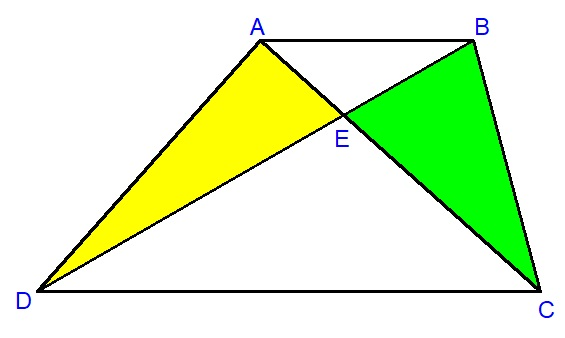Which region has a larger area?

Geometry Level 1$ABCD$ is a trapezoid with $AB||CD$. Point $E$ is the intersection of diagonals $AC$ and $BD$. Which region has a larger area? Yellow region or green region.

×